# 搞定算法与数据结构（04）：二叉树的遍历、应用及复杂度分析

## 二叉树的概念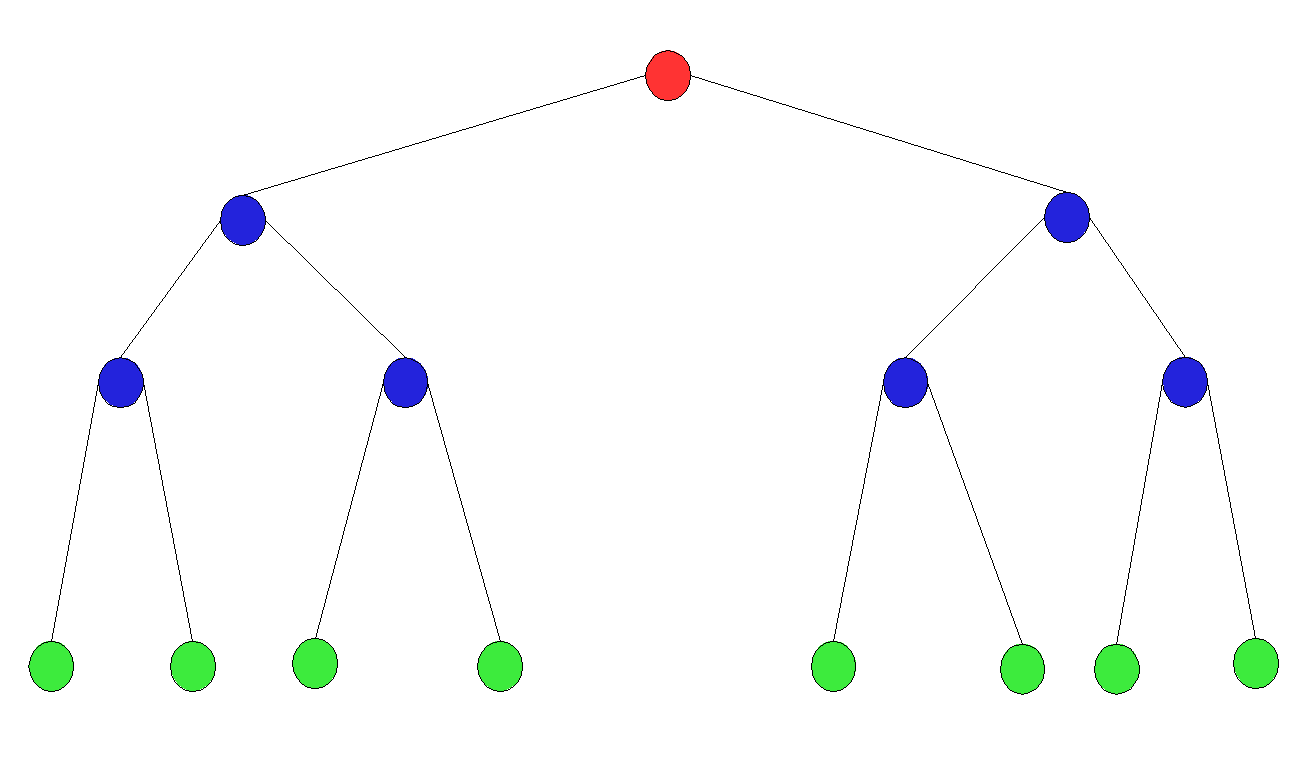• 根节点：没有父节点的节点叫做根节点，一棵树中只有一个根节点
• 兄弟节点：拥有同一个父节点的两节点，互为兄弟节点
• 叶子节点：没有子节点的节点叫做叶子节点

• 高度：距离最高层叶子节点的边数
• 深度：距离根节点的边数
• 层数：节点的深度+1
• 树的高度：根节点的高度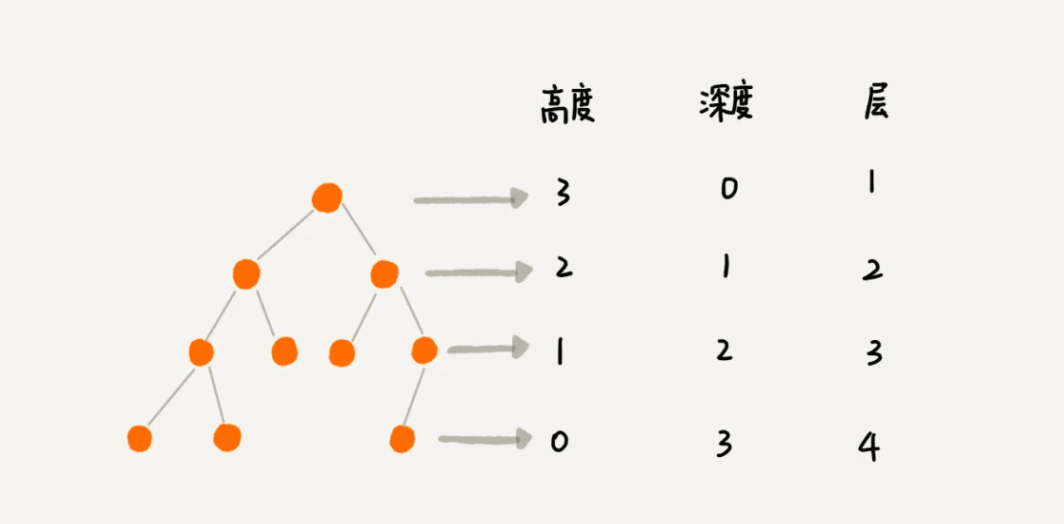• 普通二叉树
• 二叉搜索树：若二叉树每个节点的左子节点都比自己小，而右节点都比自己大，那它就是一个二叉搜索树。
• 满二叉树：一个二叉树，如果每一个层的结点数都达到最大值，则这个二叉树就是满二叉树。也就是说，如果一个二叉树的层数为K，且结点总数是(2^k) -1 ，则它就是满二叉树。
• 完全二叉树：若设二叉树的深度为h，除第 h 层外，其它各层 (1～h-1) 的结点数都达到最大个数，第 h 层所有的结点都连续集中在最左边，这就是完全二叉树。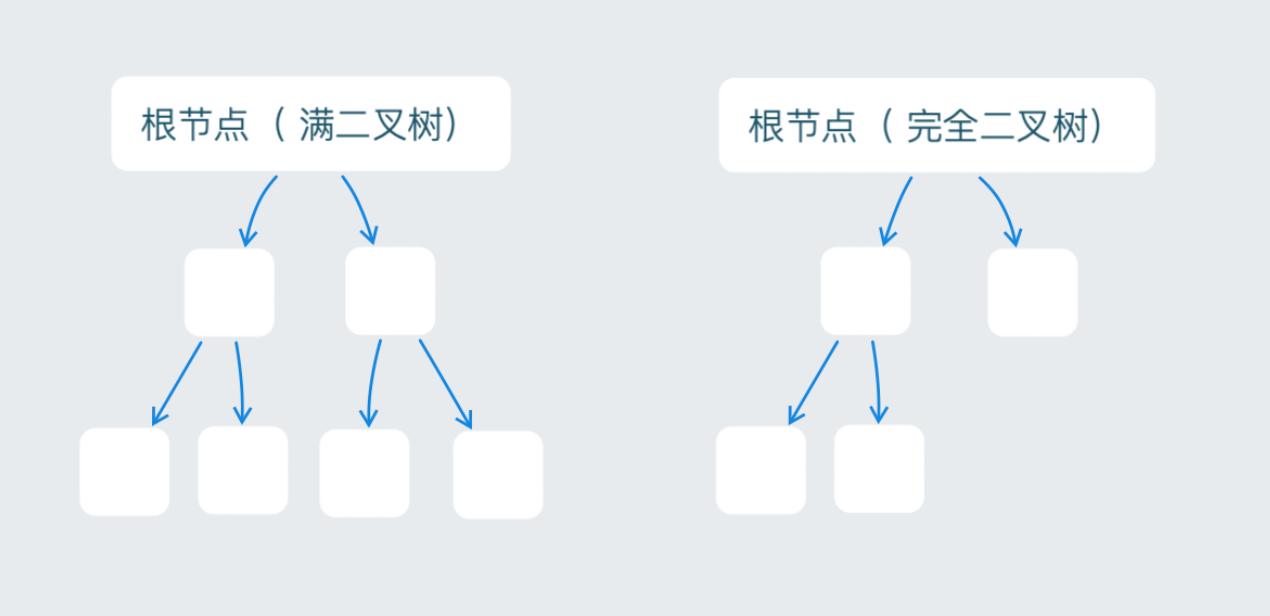## 实现二叉树深度遍历

• 先序遍历：指最先遍历节点本身，再遍历节点的左子树，最后遍历右子树的遍历方法；
• 中序遍历：指最先遍历节点的左子树，再遍历节点本身，最后遍历右子树的遍历方法，对于二叉搜索树，中序遍历可以对二叉树进行排序，时间复杂度为 O(n)，非常高效。
• 后序遍历：指最先遍历节点的左子树，再遍历右子树，最后遍历节点本身的一种遍历方法。

• 递推公式：F(node) = F(node.left) + node.value + F(node.right)
• 终止条件：node None

class TreeNode:
def __init__(self, x):
self.val = x
self.left = None
self.right = None


a = TreeNode(1)
b = TreeNode(2)
c = TreeNode(3)
d = TreeNode(4)
e = TreeNode(5)
f = TreeNode(6)
g = TreeNode(7)

a.left = b
a.right = c
b.left = d
b.right = e
c.left = f
c.right = g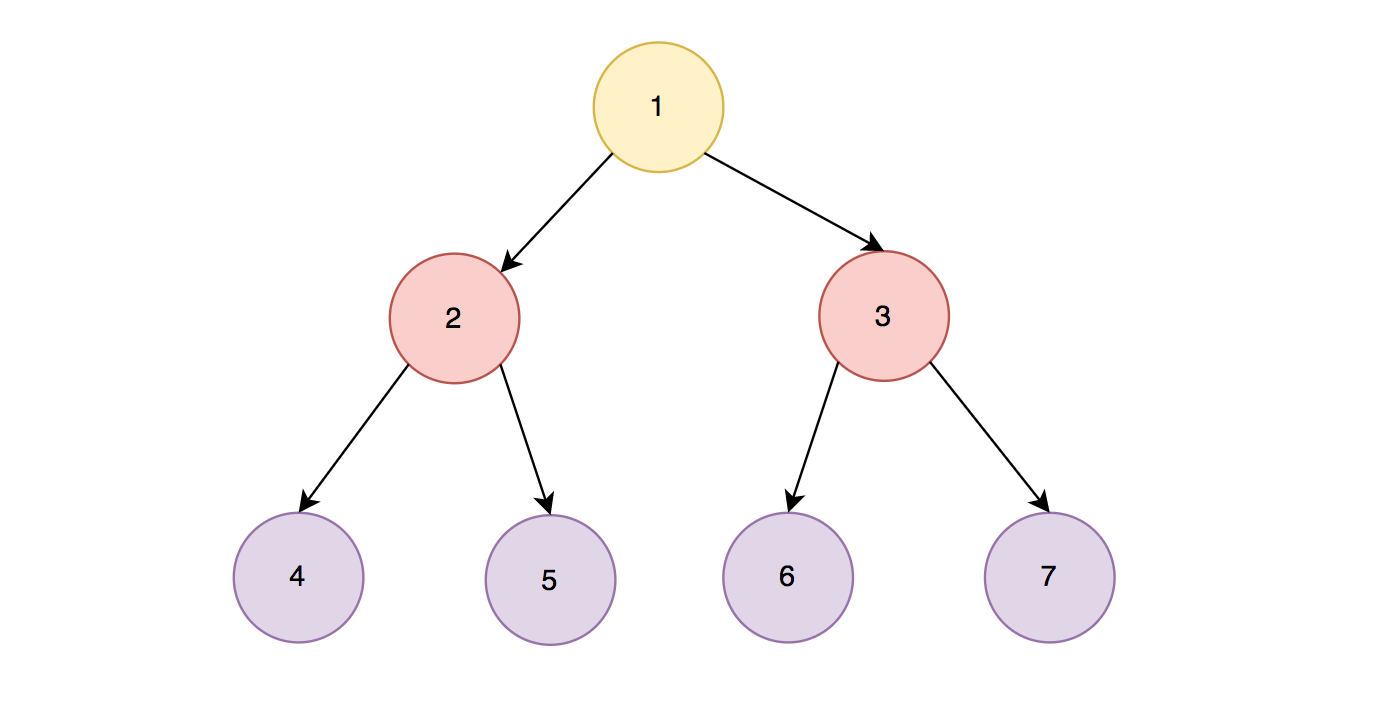# 中序打印二叉树（递归）
def in_order_traverse(node):
if node is None:
return None
in_order_traverse(node.left)
print(node.val)
in_order_traverse(node.right)


# 先序打印二叉树（递归）
def pre_order_traverse(node):
if not node:
return None
print(node.val)
pre_order_traverse(node.left)
pre_order_traverse(node.right)

# 后序打印二叉树（递归）
def post_order_traverse(node):
if node is None:
return None
post_order_traverse(node.left)
post_order_traverse(node.right)
print(node.val)


## 实现二叉树广度遍历

from queue import Queue

# 按层打印二叉树（递归）
def layer_order_traverse(node):
if node is None:
return None

queue = Queue()
queue.put(node)

while True:
n = queue.get()
if not n:
break

print(n.val)
if n.left is not None:
queue.put(n.left)

if node.right is not None:
queue.put(n.right)


## 二叉搜索树的操作

• 查找某个值
• 插入某个值
• 删除某个值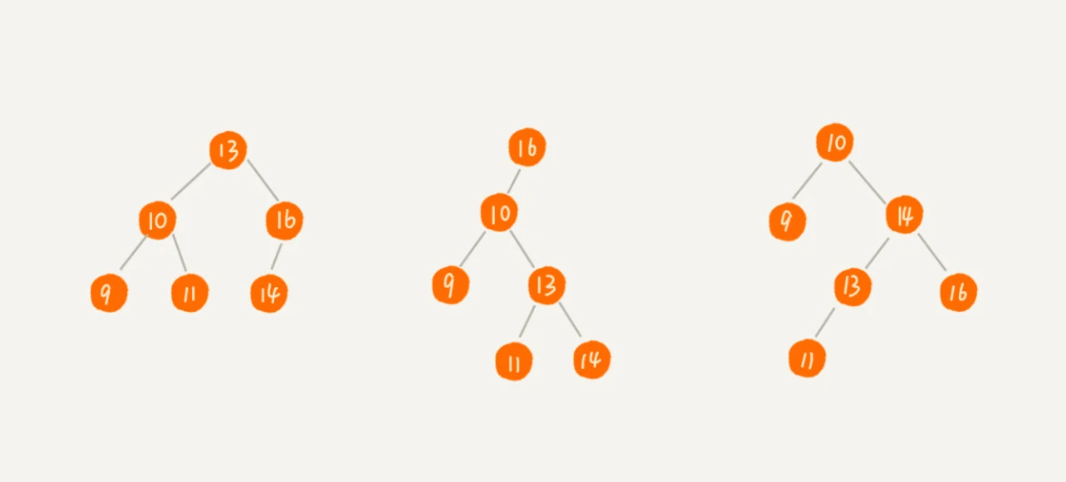### 查找某值

• 查找最大节点：直接向右遍历到底
• 查找最小节点：直接向左遍历到底
• 找出前驱节点
• 找出后继节点

• 前驱节点：比当前节点小的一堆节点里，值最大的那个节点
• 后继节点：比当前节点大的一堆节点里，值最小的那个节点

1. 若一个节点，有左子树，那么该节点的前驱节点是左子树中向右遍历到底，值最大的那个节点。比如 5 的前驱节点是 4。
2. 若一个节点，没有左子树，并且它是父节点的左节点，那么前驱节点，需要沿着父节点往上寻找。比如 2 的前驱节点是 1。
3. 若一个节点，没有左子树，并且它是父节点的右节点，那么前驱节点，就是其父节点。比如 4 的前驱节点是 3

1. 若一个节点，有右子树，那么该节点的后继节点是右子树中向左遍历到底，值最小的那个节点。比如 7 的后继节点是 8 。
2. 若一个节点，没有右子树，并且它是父节点的左节点，那么后继节点，就是其父节点。比如 8 的后继节点是 9。
3. 若一个节点，没有右子树，并且它是父节点的右节点，那么后继节点，需要沿着父节点往上寻找。比如 4 的后继节点是 5。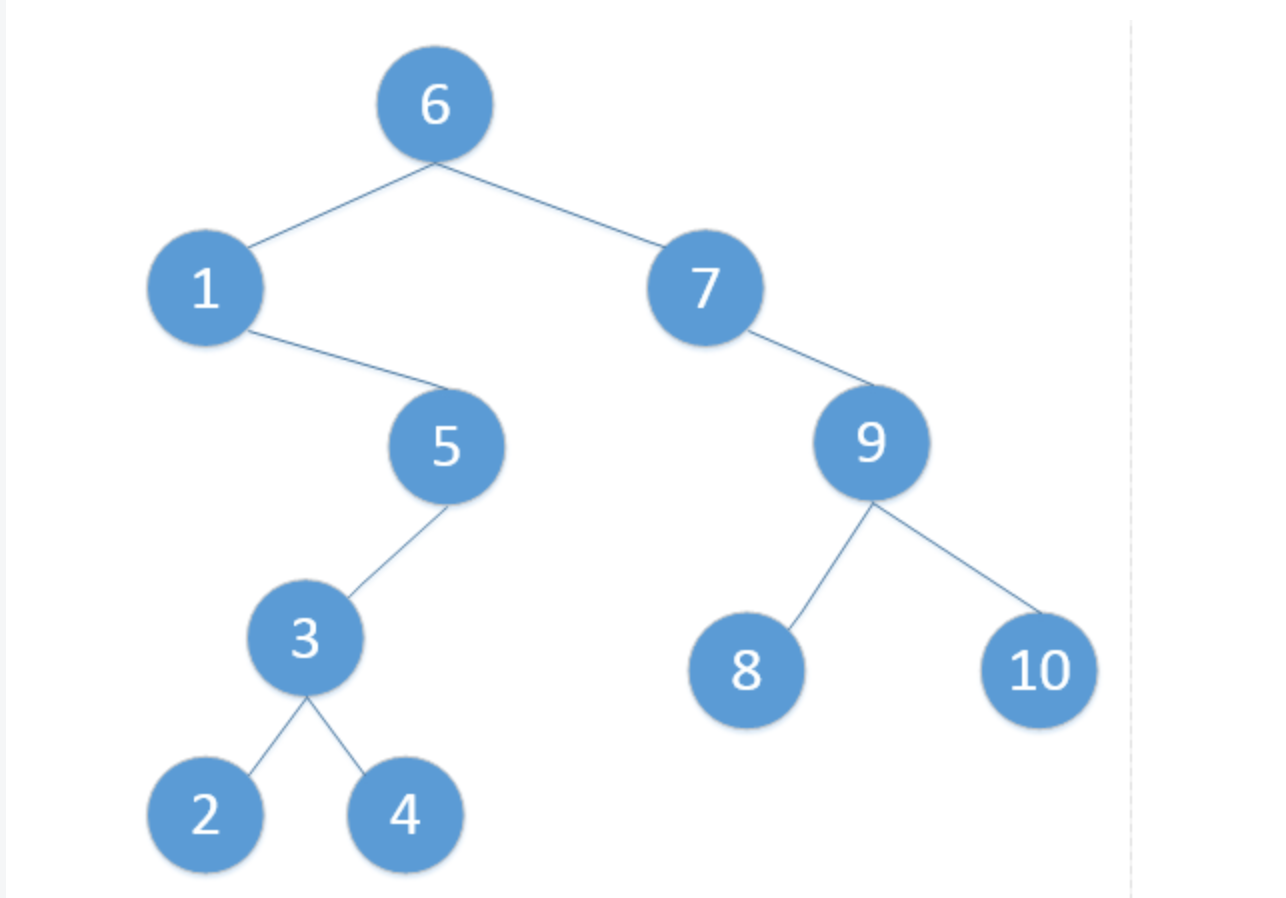### 删除某值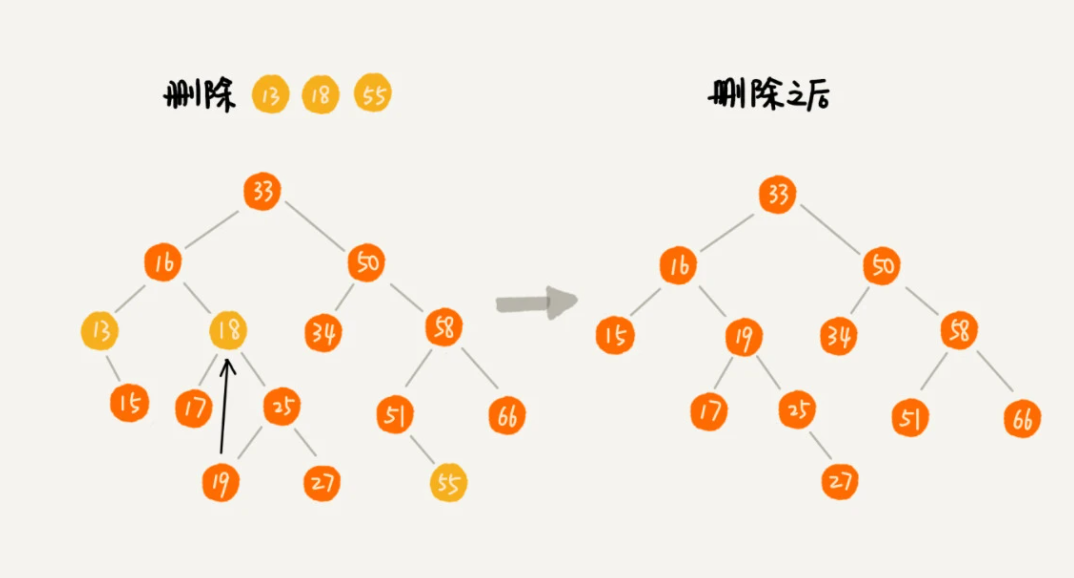## 二叉搜索树的应用## 二叉搜索树的复杂度分析

• 极度不平衡：退化成链表，如下图中第一个示例，时间复杂度为 O(n)
• 普通二叉搜索树：如下图中第二个示例，时间复杂度为 O(L)，L 为树的层数
• 完全二叉搜索树：如下图中第三个示例，时间复杂度为 O(L)，L 为树的层数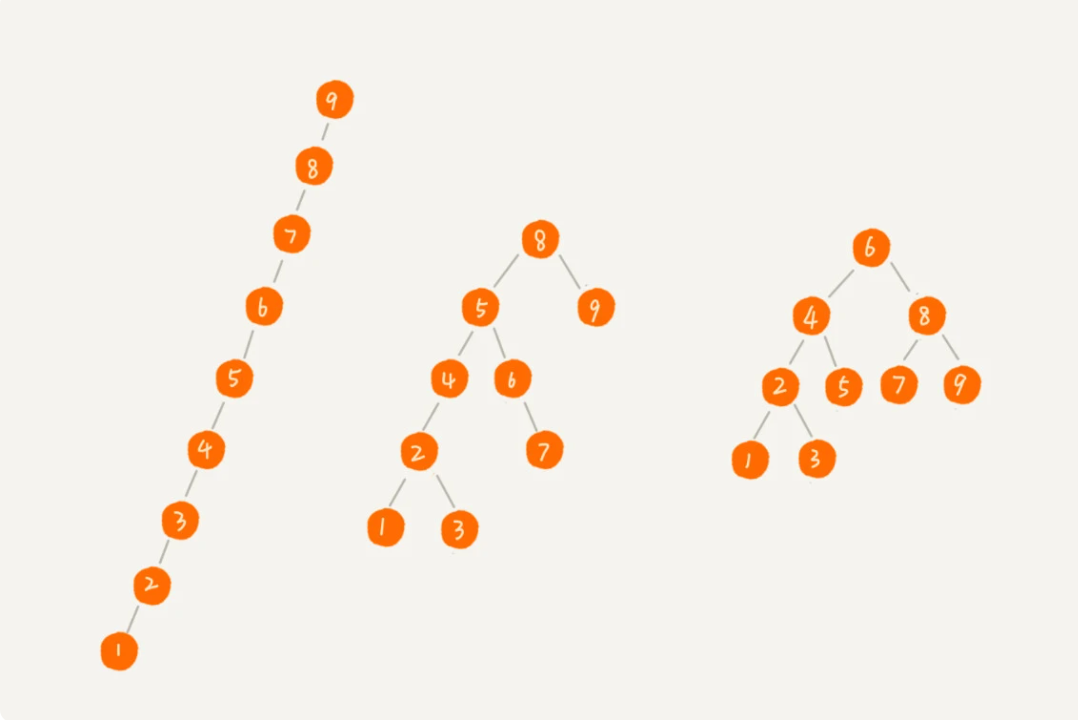L = H + 1
n >= 1 + 2^1 + 2^2 + ... + 2^(L-1) + 1
n <= 1 + 2^1 + 2^2 + ... + 2^L## 二叉树对比散列表

1. 散列表虽然查找的平均时间复杂度为 O(1)，但这仅仅是在没有散列冲突的情况下才能有如此效率，因此并不稳定。而平衡二叉搜索树的查找效率是非常稳定的，为 O(nlogn)。由于散列冲突的存在，散列表查询的常数不一定会比 O(logn) 快，并且还要加上散列函数的计算耗时。
2. 散列表数据的存储是无序的，如果要排序的话，二叉树的效率非常高，为 O(n)
3. 散列表的设计比较复杂，需要考虑的东西比较高，比如散列函数如何设计，散列冲突如何解决，扩容、缩容等等